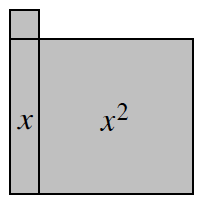### Home > CC1MN > Chapter 8 > Lesson 8.2.5 > Problem8-115

8-115.

Sketch the algebra-tile shape at right on your paper. Write an expression for the perimeter of the shape. Then find the perimeter for each of the given values of $x$.

Find the perimeter as you did in problems 6-96 and 6-106, including $x$ as part of the expression.

$P = 4x + 4$1. $x = 7 \text{ units}$

Substitute $7$ for $x$ in the expression of the perimeter.

Evaluate the expression.
$4(7) + 4 = ?$

$32$ units

1. $x = 5.5\text{ units}$

• Substitute $5.5$ for $x$ in the expression of the perimeter and evaluate.

$26$ units

1. $x = \frac { 7 } { 3 }\text{ units}$

• See part (a) or (b).